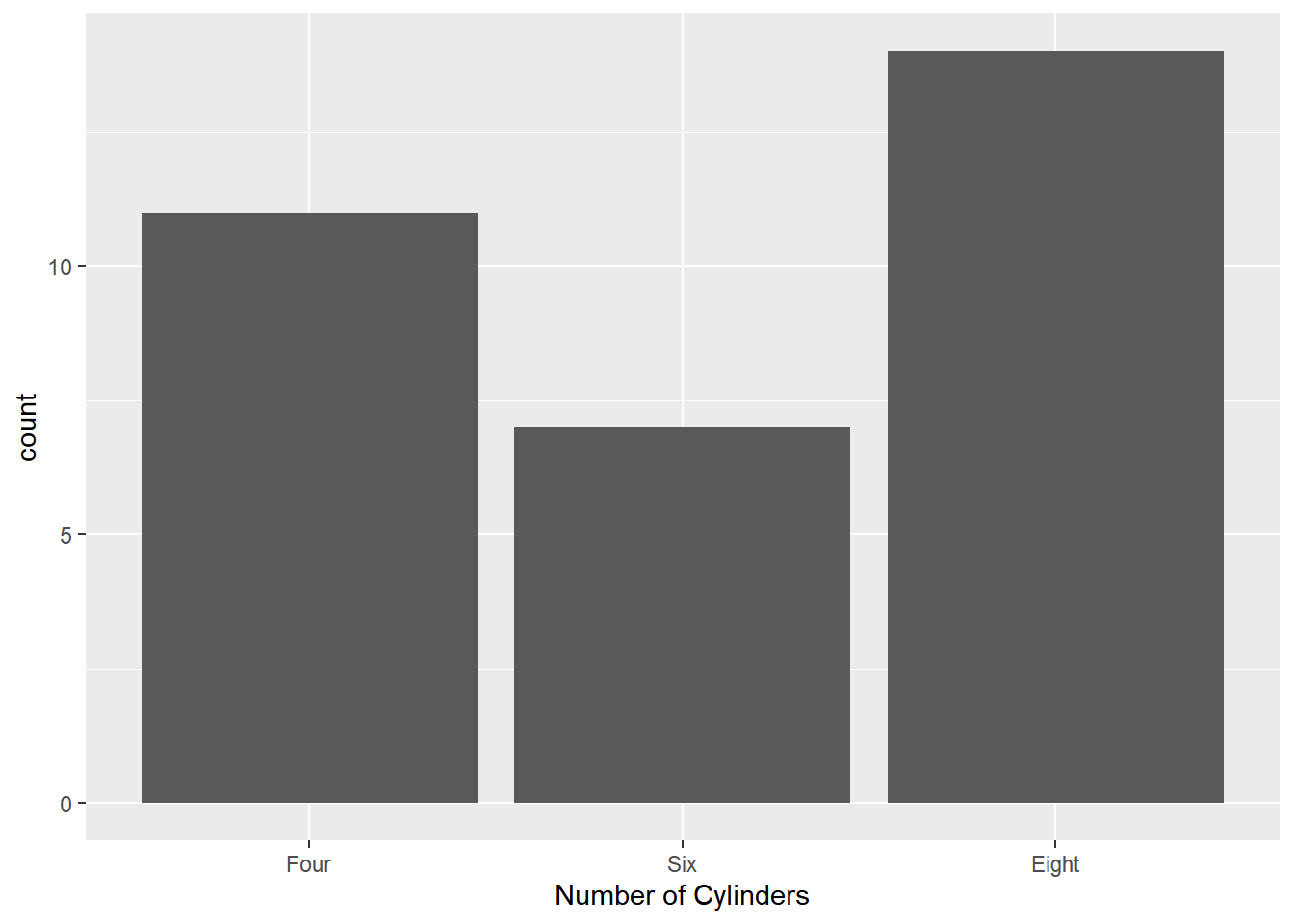# Chapter 11 Modify Axis

In this chapter, we will learn how to modify the X and Y axis using the following functions:

• Continuous Axis
• `scale_x_continuous()`
• `scale_y_continuous()`
• Discrete Axis
• `scale_x_discrete()`
• `scale_y_discrete()`

## 11.1 Continuous Axis

If the X and Y axis represent continuous data, we can use `scale_x_continuous()` and `scale_y_continuous()` to modify the axis. They take the following arguments:

• name
• limits
• breaks
• labels
• position

Let us continue with the scatter plot we have used in previous chapter.

``````ggplot(mtcars) +
geom_point(aes(disp, mpg))``````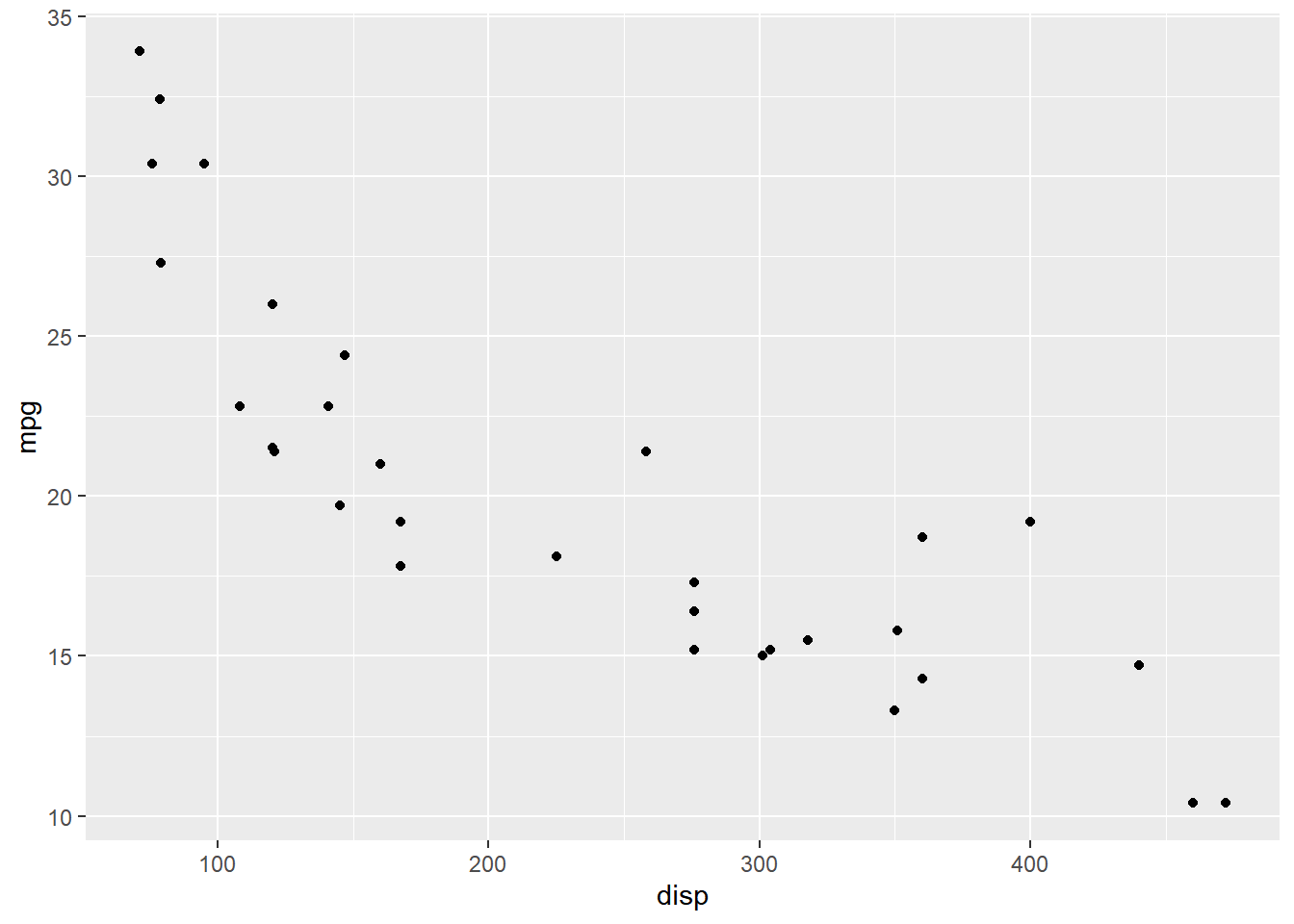The `name` argument is used to modify the X axis label. In the below example, we change the X axis label to `'Displacement'`. In previous chapters, we have used `xlab()` to work with the X axis label.

``````ggplot(mtcars) +
geom_point(aes(disp, mpg)) +
scale_x_continuous(name = "Displacement")``````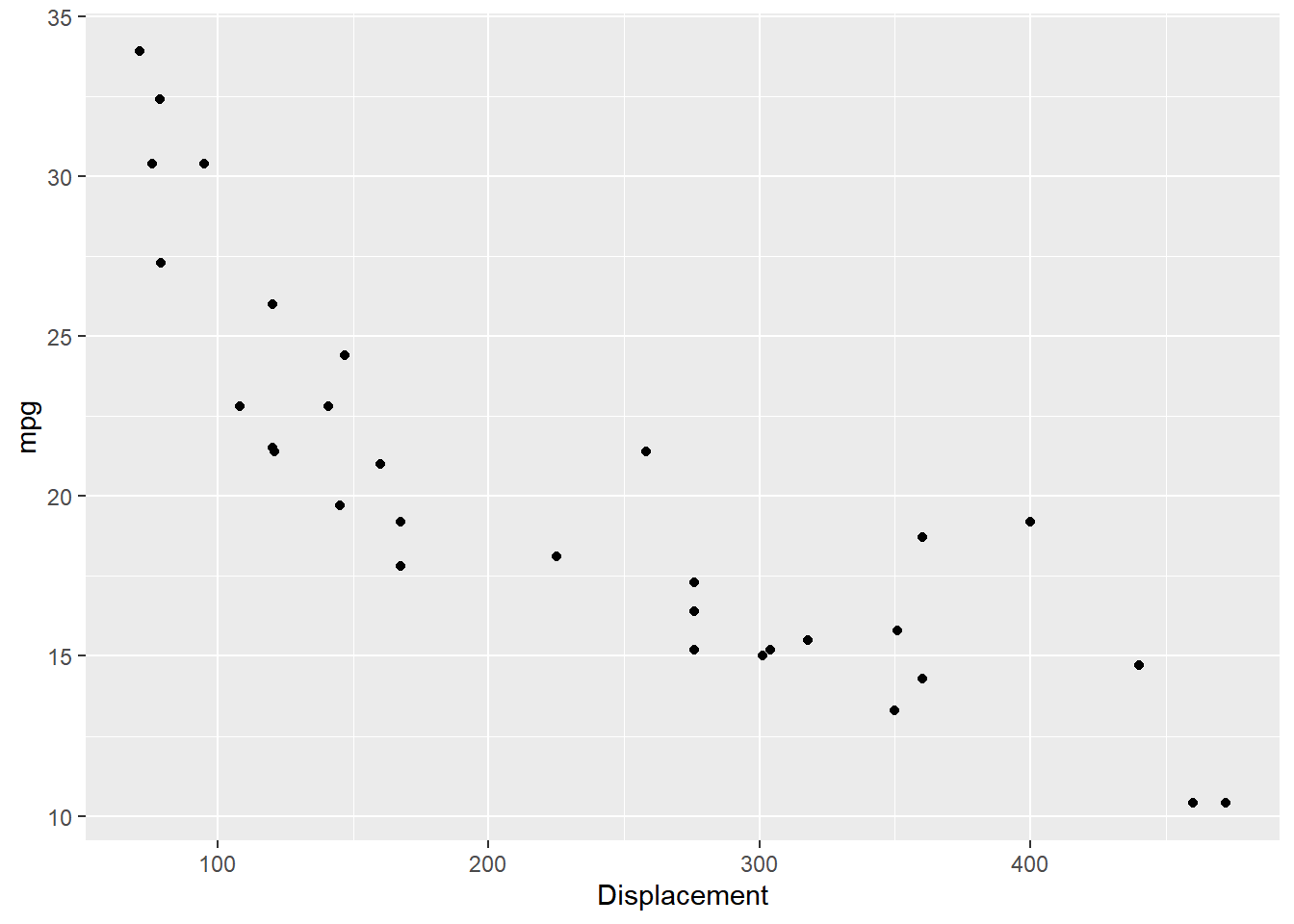To modify the range, use the `limits` argument. It takes a vector of length 2 i.e. 2 values, the lower and upper limit of the range. It is an alternative for `xlim()`.

``````ggplot(mtcars) +
geom_point(aes(disp, mpg)) +
scale_x_continuous(limits = c(0, 600))``````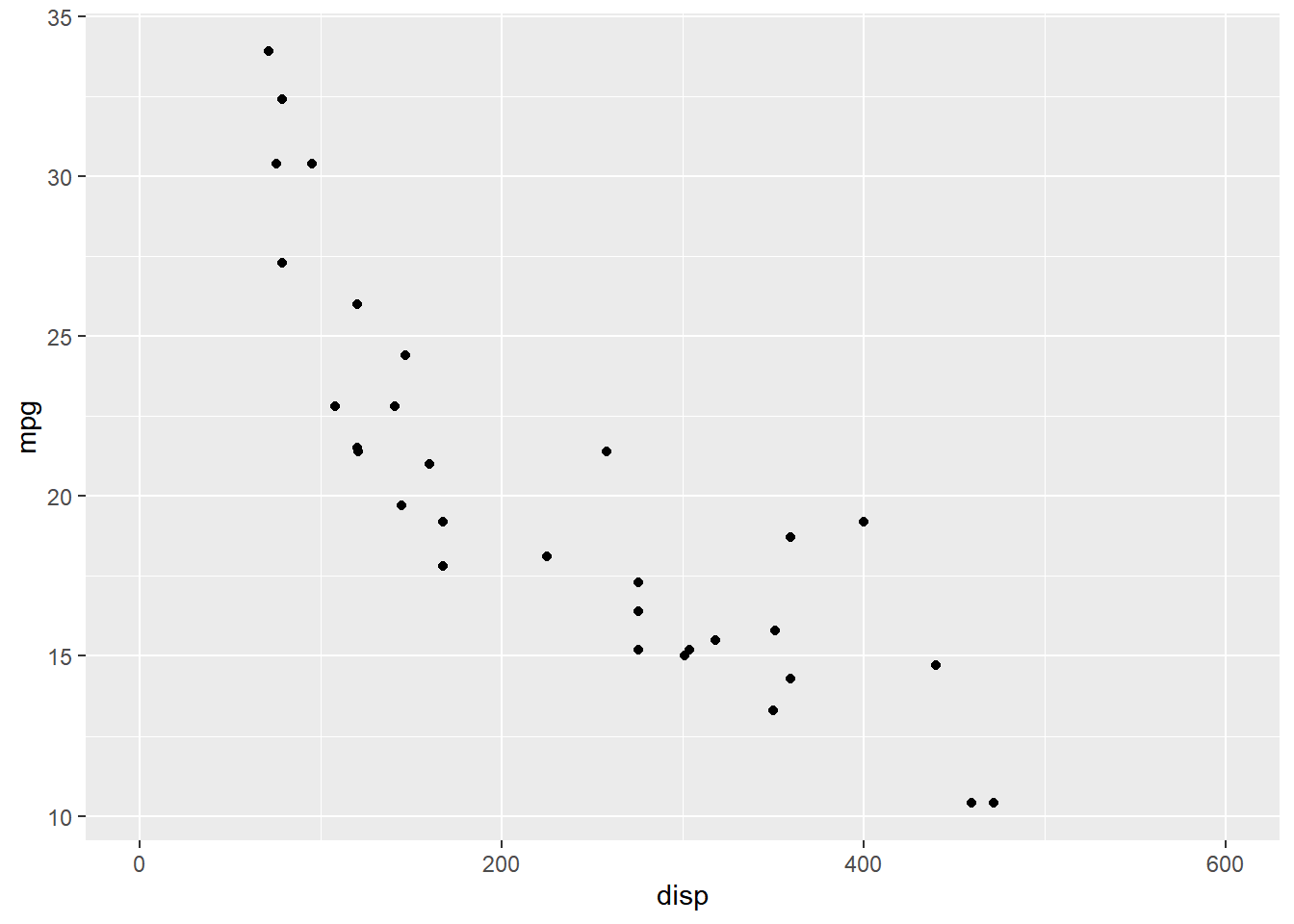In the above plot, the ticks on the X axis appear at `0`, `200`, `400` and `600`. Let us say we want the ticks to appear more closer i.e. the difference between the tick should be reduced by `50`. The `breaks` argument will allow us to specify where the ticks appear. It takes a numeric vector equal to the length of the number of ticks.

``````ggplot(mtcars) +
geom_point(aes(disp, mpg)) +
scale_x_continuous(breaks = c(150, 300, 450))``````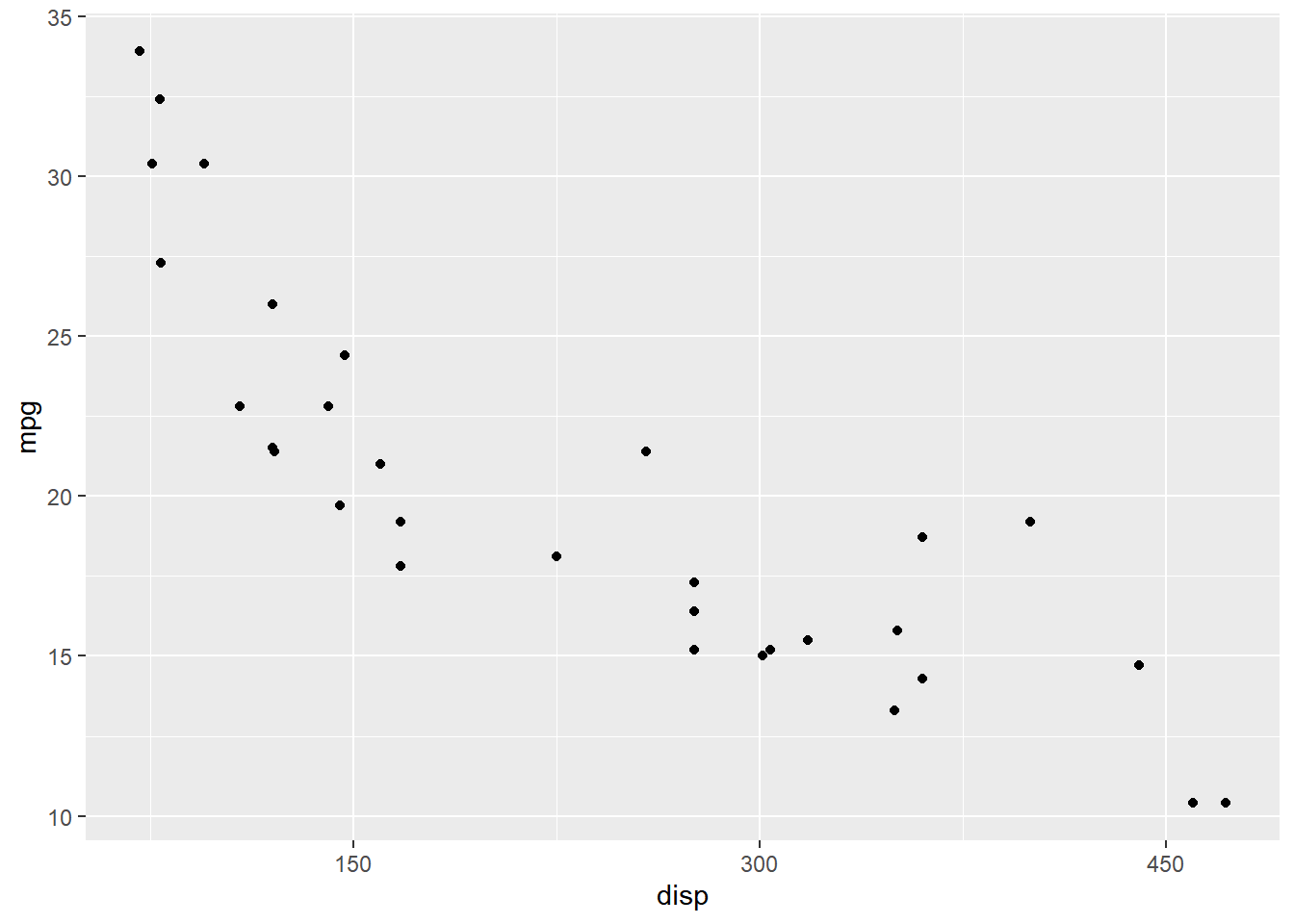We can change the tick labels using the `labels` argument. In the below example, we use words instead of numbers. When adding labels, we need to ensure that the length of the `breaks` and `labels` are same.

``````ggplot(mtcars) +
geom_point(aes(disp, mpg)) +
scale_x_continuous(breaks = c(150, 300, 450),
labels = c('One Hundred Fifty', 'Three Hundred', 'Four Hundred Fifity'))``````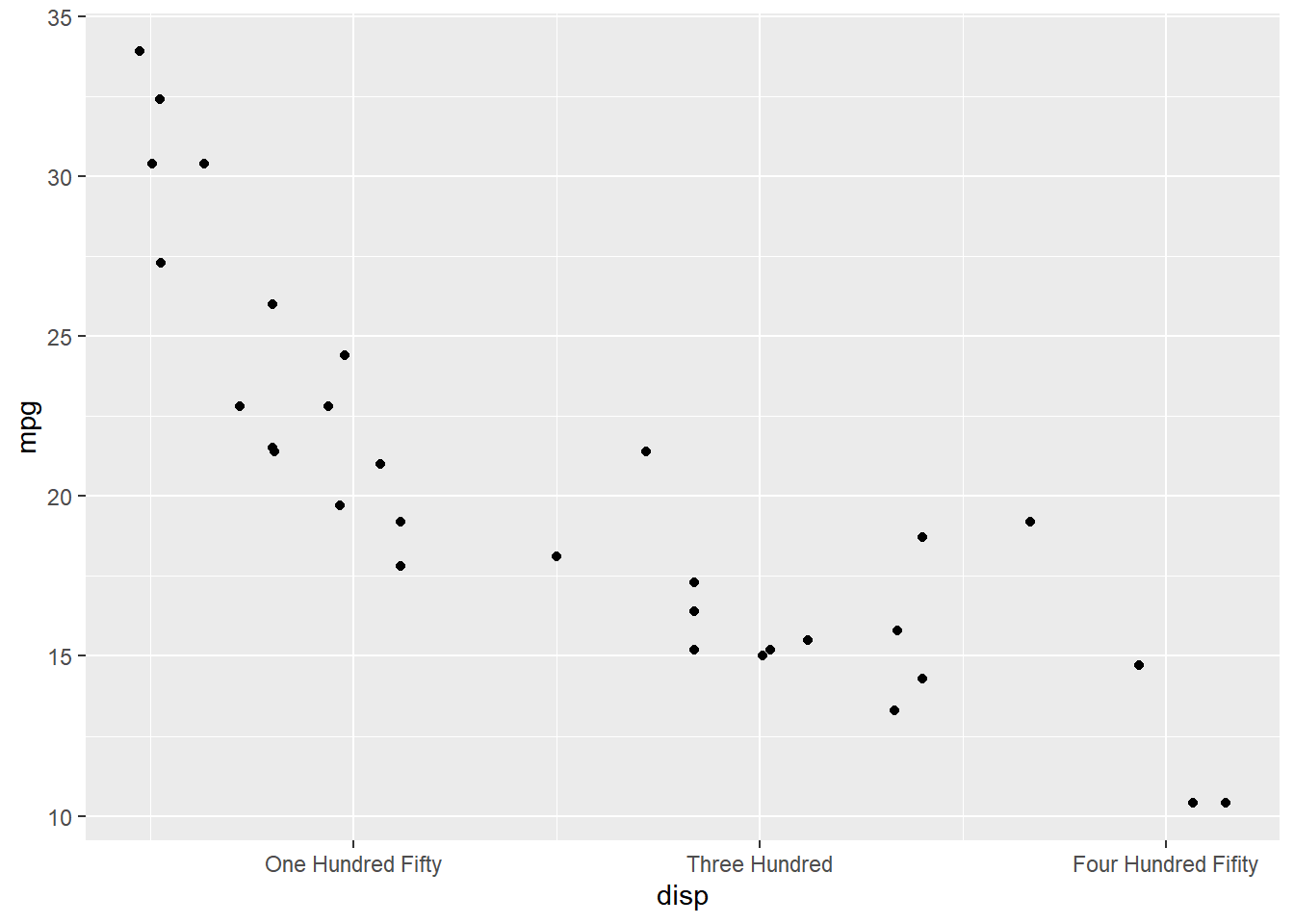The position of the axes can be changed using the `position` argument. In the below example, we can move the axes to the top of the plot by supplying the value `'top'`.

``````ggplot(mtcars) +
geom_point(aes(disp, mpg)) +
scale_x_continuous(position = 'top')``````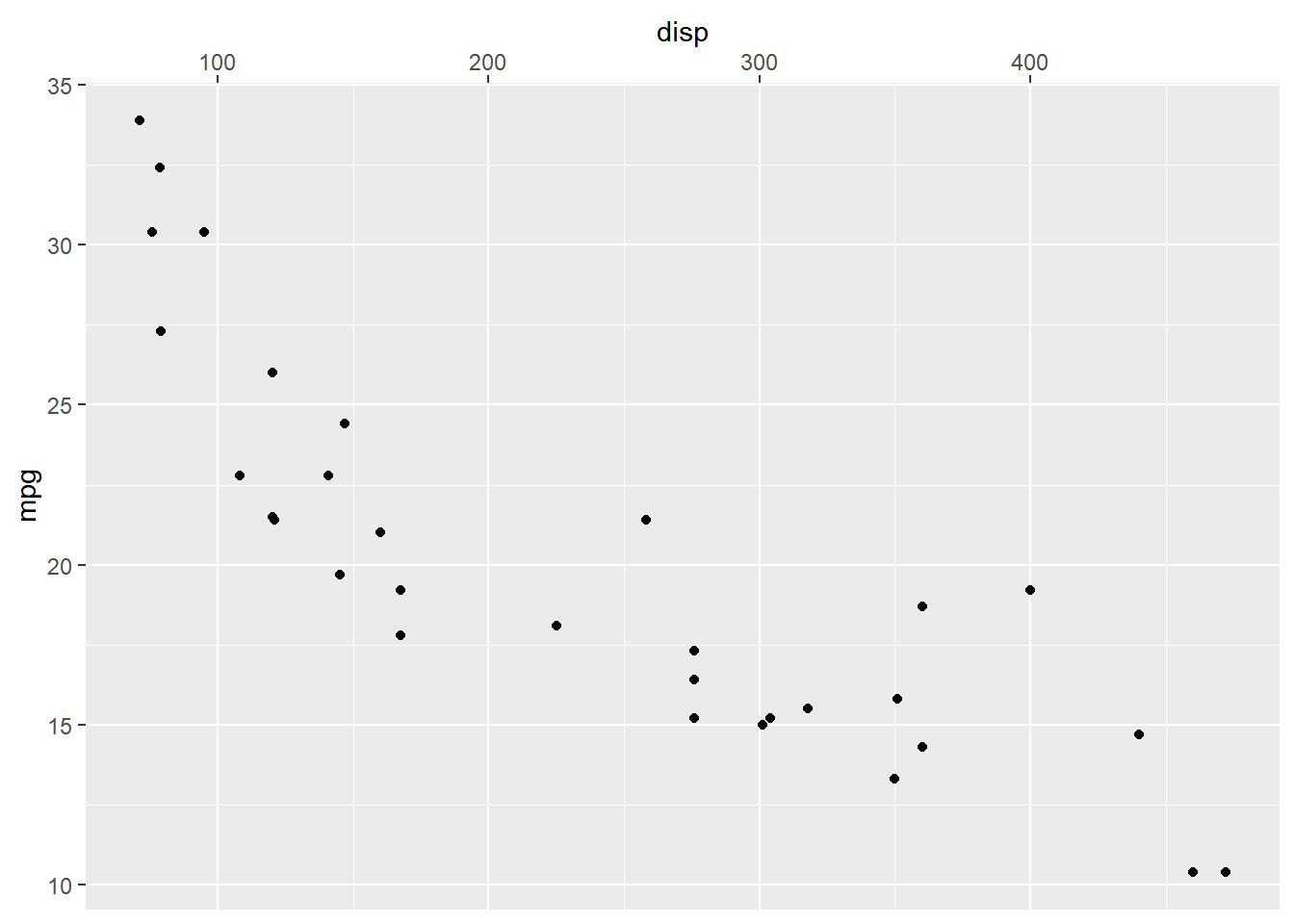### 11.1.1 Putting it all together..

``````ggplot(mtcars) + geom_point(aes(disp, mpg)) +
scale_x_continuous(name = "Displacement", limits = c(0, 600),
breaks = c(0, 150, 300, 450, 600), position = 'top',
labels = c('0', '150', '300', '450', '600'))``````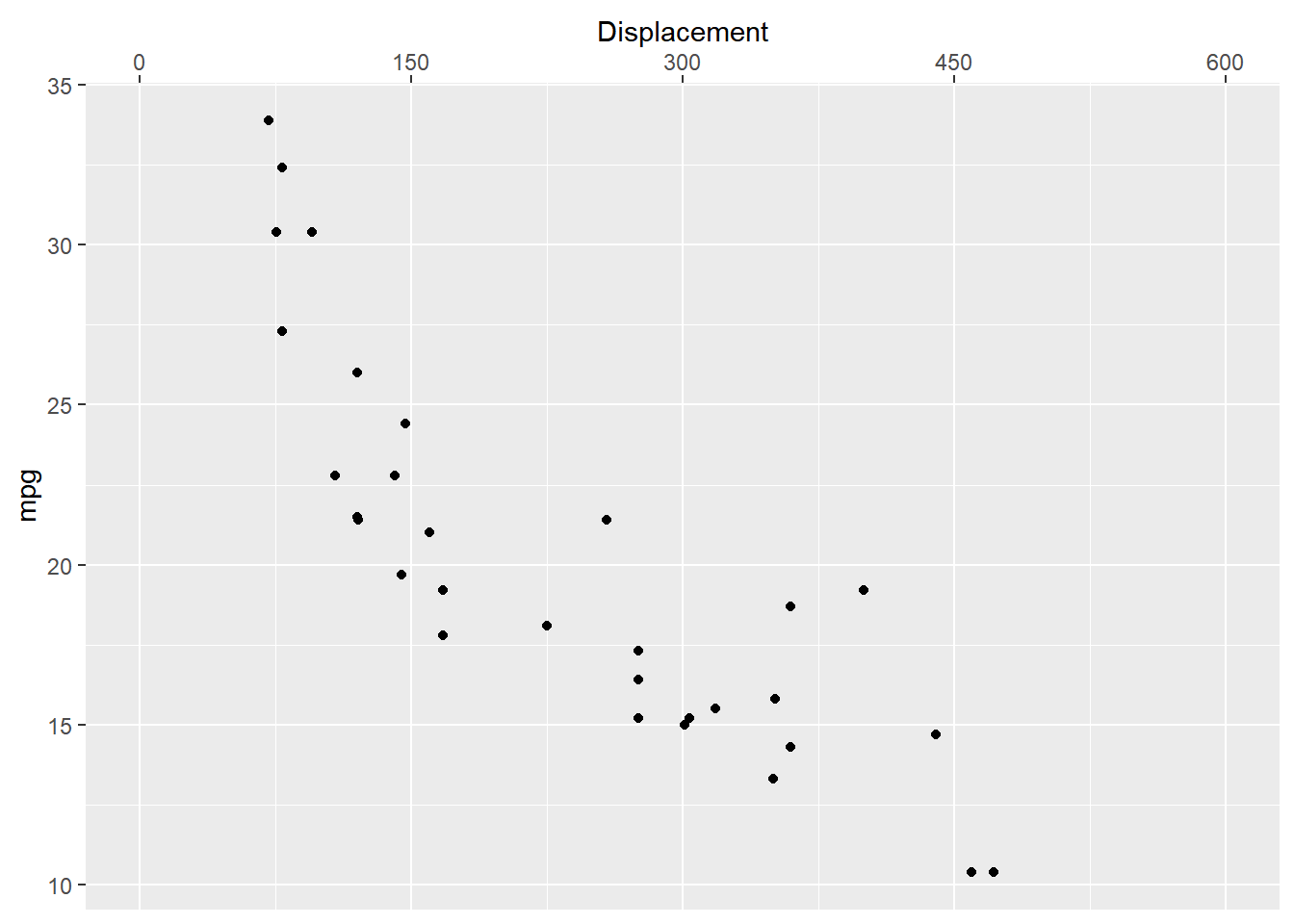### 11.1.2 Y Axis - Continuous

``````ggplot(mtcars) + geom_point(aes(disp, mpg)) +
scale_y_continuous(name = "Miles Per Gallon", limits = c(0, 45),
breaks = c(0, 15, 30, 45), position = 'right',
labels = c('0', '15', '30', '45'))``````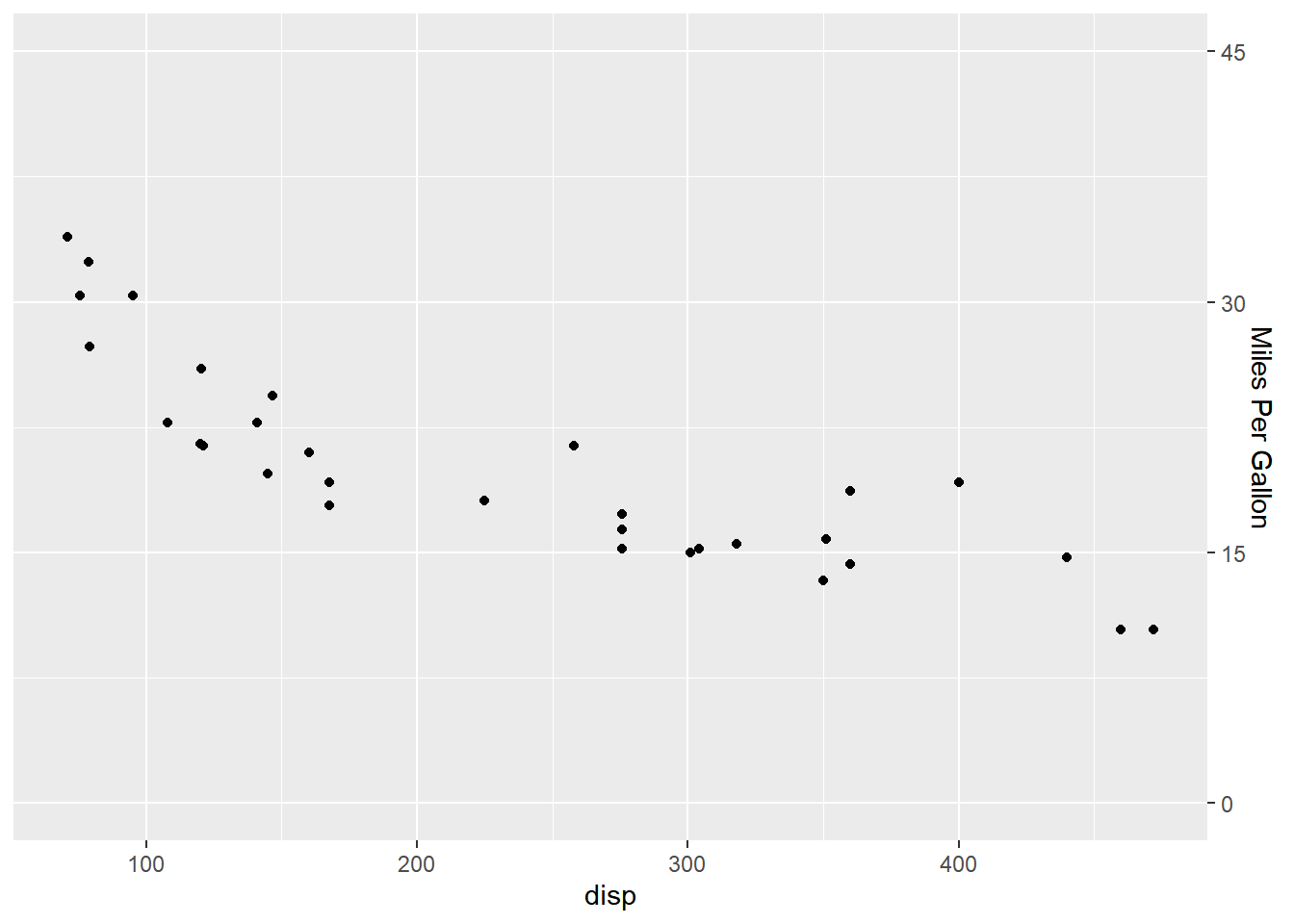## 11.2 Discrete Axis

If the X and Y axis represent discrete or categorical data, `scale_x_discrete()` and `scale_y_discrete()` can be used to modify them. They take the following arguments:

• name
• labels
• breaks
• position

The above options serve the same purpose as in the case of continuous scales.

### 11.2.1 Axis Label

``````ggplot(mtcars) +
geom_bar(aes(factor(cyl))) +
scale_x_discrete(name = "Number of Cylinders")``````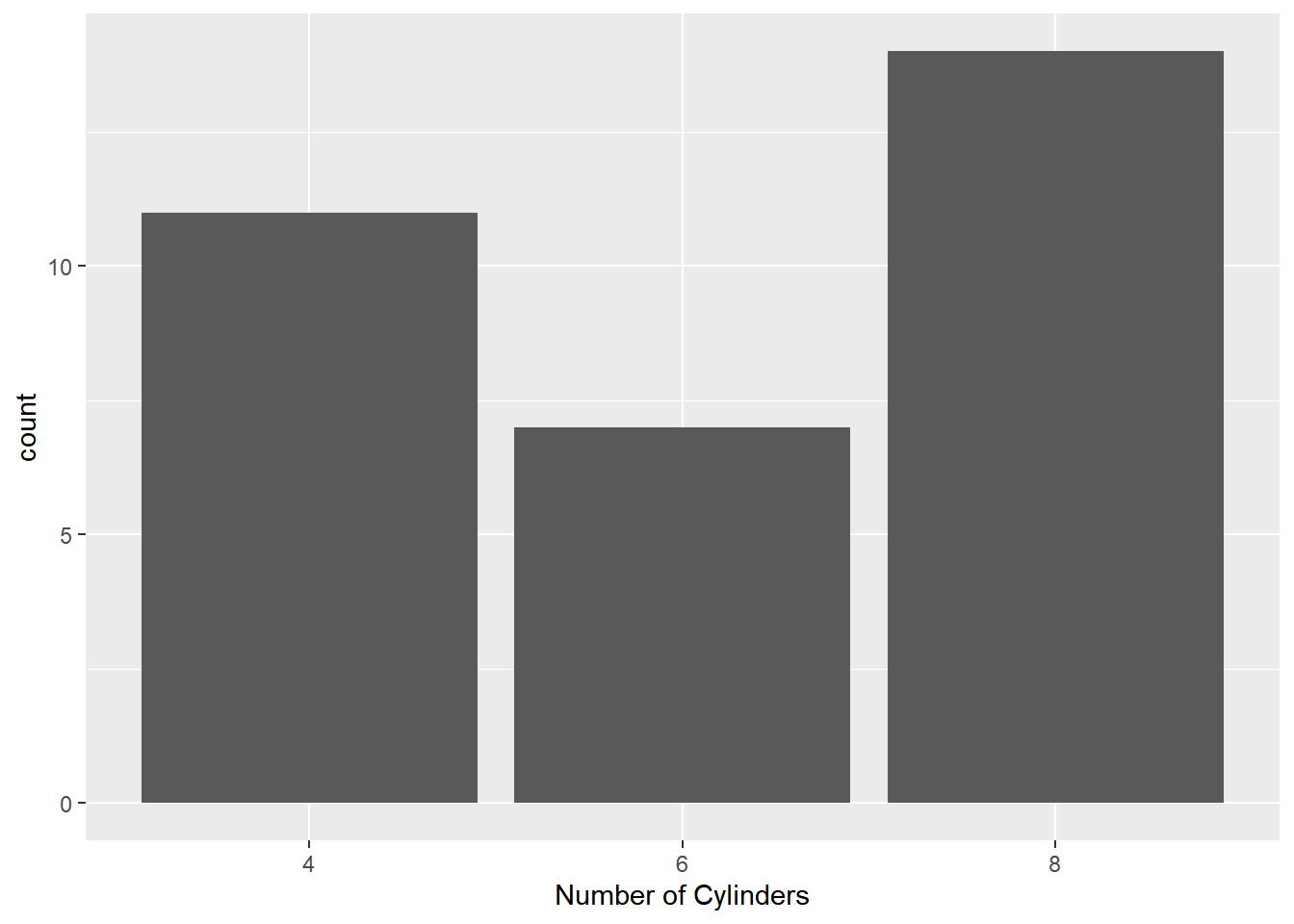### 11.2.2 Axis Tick Labels

``````ggplot(mtcars) +
geom_bar(aes(factor(cyl))) +
scale_x_discrete(labels = c("4" = "Four", "6" = "Six", "8" = "Eight"))``````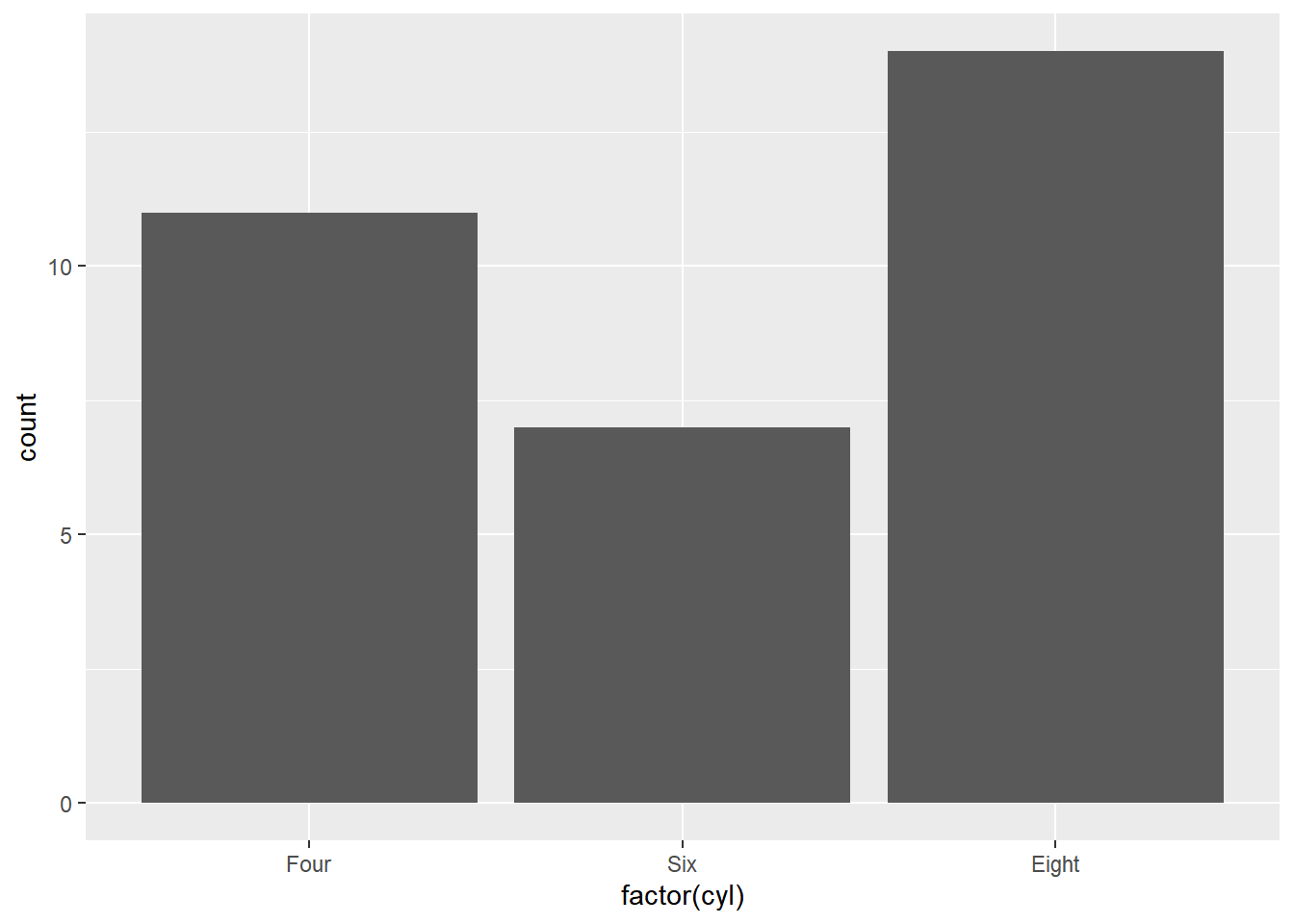### 11.2.3 Axis Breaks

``````ggplot(mtcars) +
geom_bar(aes(factor(cyl))) +
scale_x_discrete(breaks = c("4", "6", "8"))``````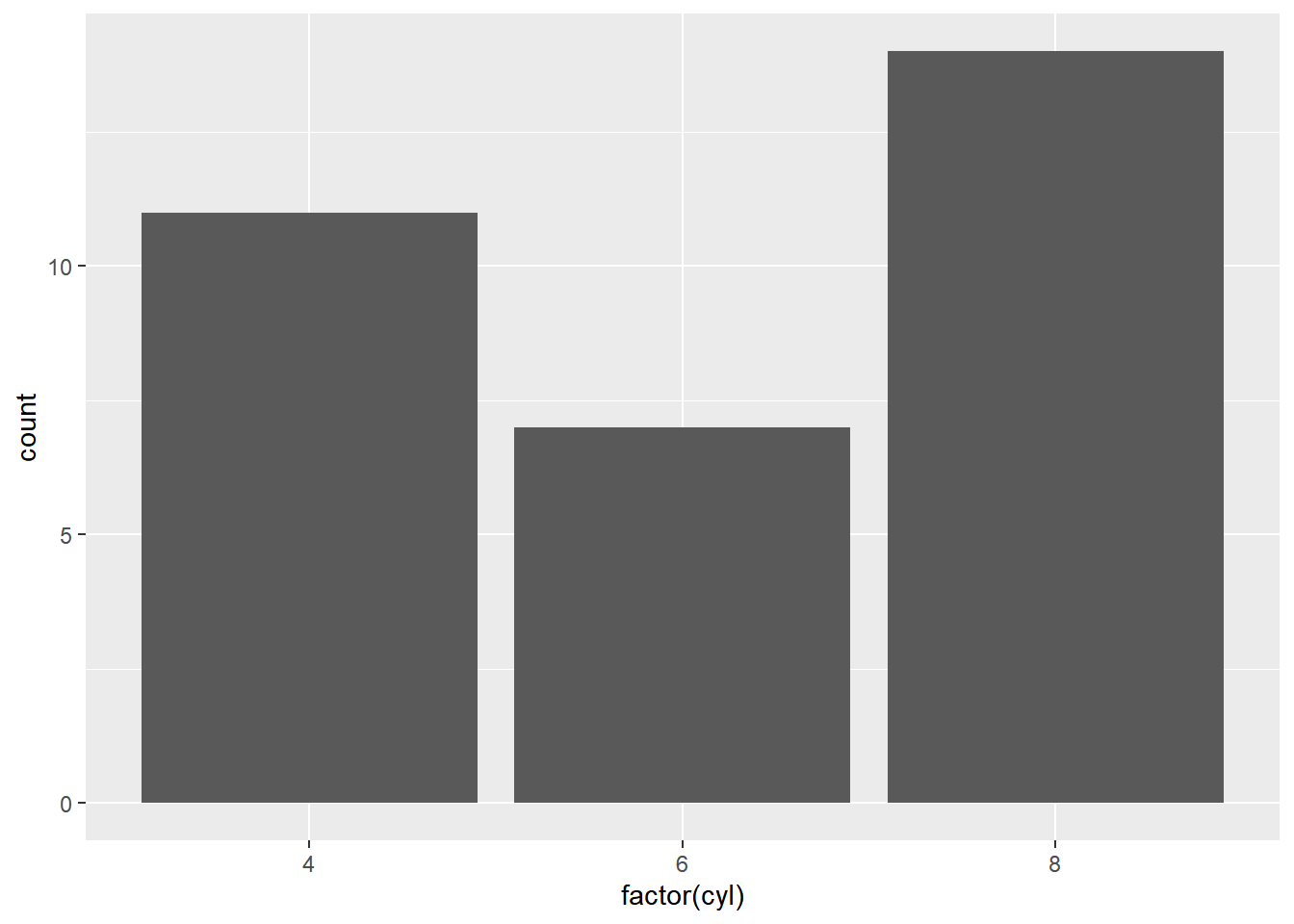### 11.2.4 Axis Position

``````ggplot(mtcars) +
geom_bar(aes(factor(cyl))) +
scale_x_discrete(position = 'bottom')``````## 11.3 Putting it all together…

``````ggplot(mtcars) + geom_bar(aes(factor(cyl))) +
scale_x_discrete(name = "Number of Cylinders",
labels = c("4" = "Four", "6" = "Six", "8" = "Eight"),
breaks = c("4", "6", "8"), position = "bottom")``````